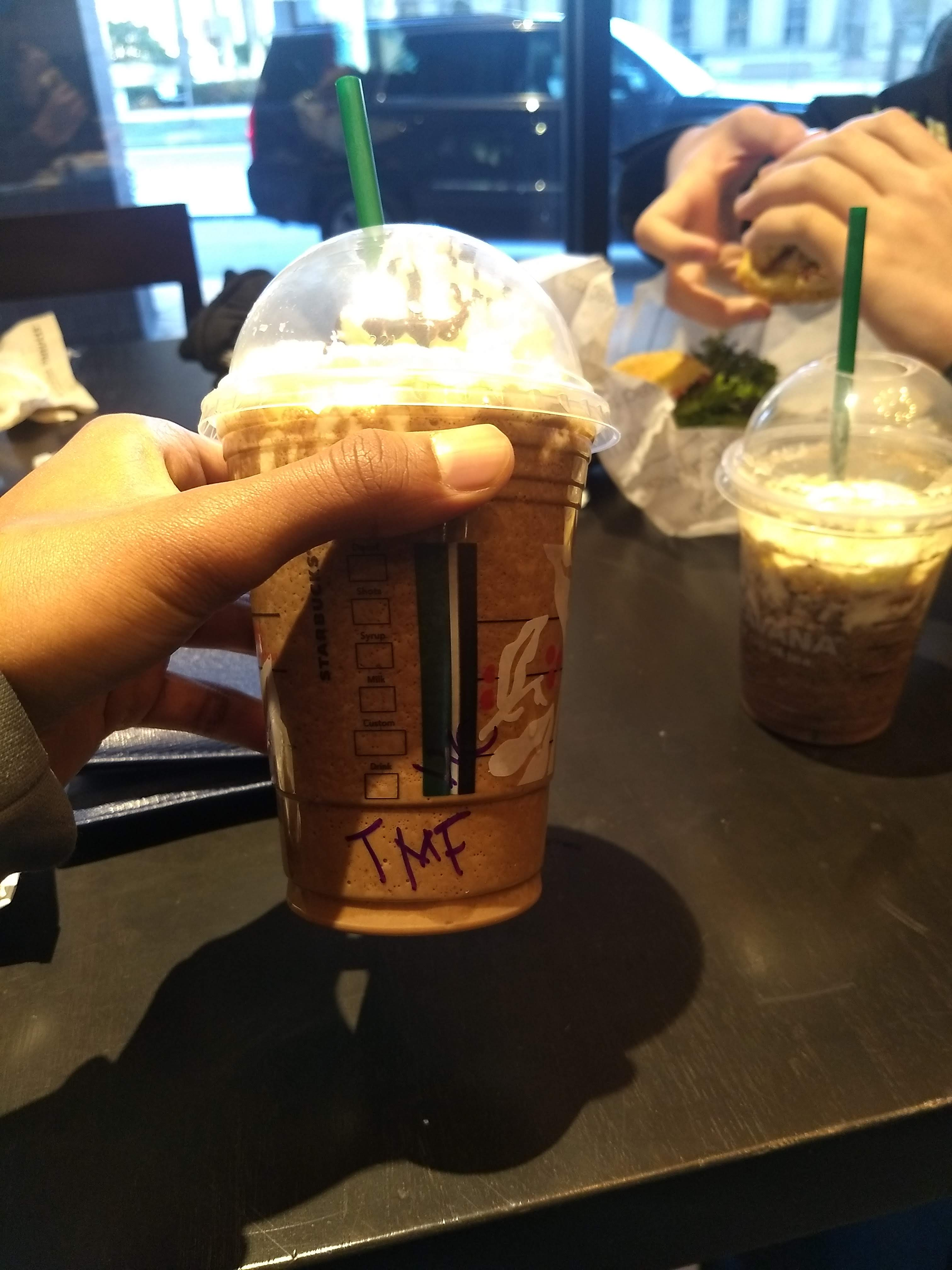I'm a first-year graduate student at Harvard. I'm broadly interested in algebraic topology and arithmetic geometry, but my interests are constantly changing. Outside of math, I spend a bunch of time playing the drums, listening to instrumental prog metal, biking, and playing basketball. I was an undergraduate at MIT, where I majored in math (course 18) and minored in physics (course 8). Email address: sdevalapurkar[at]math[dot]harvard[dot]edu. Apparently I've got an office in SC 333f, but it seems unlikely that you'd find me there this year. CV: [pdf] I'm also on MathOverflow. Here are the courses that I've taken, as well as a blog (which I have been horrible about updating).

## Writings

### Some projects

• Quantization and Koszul duality.
Discusses the theory of deformation quantization from a Koszul dual perspective, roughly allowing us to view deformation quantization as the datum of an $$S^1$$-action. In characteristic $$p$$, this can be refined further: restricted deformation quantization can roughly be viewed as the datum of an $$S^1$$-action along with a cyclotomic structure. Applications to the deformation theory of schemes are discussed.

• On the James and Hilton-Milnor Splittings, and the metastable EHP sequence (2019), joint with Peter Haine. Last update: November 2020. arXiv.
Studies the James and Hilton-Milnor splittings and the metastable EHP sequences in higher category theory; this allows a generalization of the James and Hilton-Milnor splittings to motivic spaces over any base scheme. Two proofs of the metastable EHP sequence are provided; one is new and non-calculational, and (essentially) only utilizes the Blakers-Massey theorem.

• Higher chromatic Thom spectra via unstable homotopy theory (2020). Last update: June 2020. arXiv.
Proves that a conjecture in unstable homotopy theory related to the Cohen-Moore-Neisendorfer theorem, coupled with a conjecture about the $$\mathbf{E}_3$$-centrality of a certain element in the homotopy of Ravenel’s $$X(n)$$ spectra, leads to a construction of truncated Brown-Peterson spectra, $$\mathrm{bo}$$, and $$\mathrm{tmf}$$ as Thom spectra (albeit not over the sphere); this is a higher chromatic analogue of the Hopkins-Mahowald theorem constructing $$\mathrm{H}\mathbf{F}_p$$ as a Thom spectrum. The construction of tmf via this method is used to show that these conjectures imply that the string orientation $$\mathrm{MString} \to \mathrm{tmf}$$ splits.
Last update: fixed some embarassing typos.

• The Ando-Hopkins-Rezk orientation is surjective (2019). Last update: January 2020. arXiv.
Shows that the Ando-Hopkins-Rezk orientation $$\mathrm{MString} \to \mathrm{tmf}$$ is surjective on homotopy. This is done by constructing an $$\mathbf{E}_1$$-ring $$B$$ with an $$\mathbf{E}_1$$-map $$B\to \mathrm{MString}$$, and showing that the composite $$B \to \mathrm{MString} \to \mathrm{tmf}$$ is surjective on homotopy. File for generating the Adams chart via Hood Chatham’s Ext calculator.

• Hodge theory for elliptic curves and the Hopf element $$\nu$$ (2019). Last update: April 2020. arXiv.
Shows that the sheaf on the moduli stack of elliptic curves associated to $$\nu$$ is isomorphic to the de Rham cohomology of the universal elliptic curve. Uses this to study the $$\mathbf{E}_1$$-quotient of $$\mathrm{tmf}$$ by $$\nu$$ by relating it to the moduli stack of elliptic curves with a chosen splitting of the Hodge filtration; this results in a calculation of the resulting descent/Adams-Novikov spectral sequence. Latest version fixes a small calculational error (results remain unchanged).

• Roots of unity in $$K(n)$$-local rings (2017). Published in Proc. Amer. Math. Soc. Last update: November 2019. arXiv.
Shows that for $$n>0$$, any $$K(n)$$-local $$H_\infty$$-ring $$R$$ with a primitive $$p^k$$-th root of unity in $$\pi_0 R$$ is trivial. This implies that the Lubin-Tate tower does not lift to a tower of derived stacks over Morava E-theory.

• Two books on algebraic topology, notes from course taught by Haynes Miller. Last update: April 2018.
• The Dieudonn’e modules and Ekedahl-Oort types of Jacobians of hyperelliptic curves in odd characteristic (2017), with John Halliday. Last update: December 2017. arXiv. Provides explicit formulae for the Frobenius and Verschiebung acting on the mod p Dieudonn’e module of the Jacobian of a hyperelliptic curve, when p is an odd prime. These formulae are used to settle some questions posed by Glass and Pries from 2004. The code used is available at this github repository.

• The Lubin-Tate stack and Gross-Hopkins duality (2017). Last update: July 2018. arXiv.
Uses derived algebraic geometry to provide proofs and generalizations of some duality phenomena in K(n)-local stable homotopy theory. (Fixed some typos and technical errors, and some results on K(n)-local Spanier-Whitehead self-duality of higher real K-theories.)

• Talbot proceedings: Obstruction theory for structured ring spectra (2017), joint with Eva Belmont et. al. Last update: September 2017.
The proceedings from the Talbot workshop on obstruction theory for structured ring spectra, which took place from May 21-27, 2017.

• The importance of theoretical research (2018). Article published in The Tech.